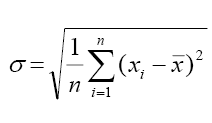# How To Find Standard Deviation From Variance

Standard deviation calculates the dispersion of a dataset relative to its mean. A standard deviation is a useful tool in investing and trading strategies. If the data points are away from the mean, there is a higher deviation within the data set. Hence, the more spread out the data, the greater the standard deviation. Standard deviation cannot be negative. Mean is the average of a given set of observations. Variance is a measure of central dispersion. It is the average of the squared difference from the mean. In this article, we will discuss how to find the standard deviation from variance.

## What is Standard Deviation?

Standard deviation calculates the dispersion of a dataset relative to its mean.

The average of the squared differences from the mean is known as the variance. Variance is denoted by σ2.

## How to calculate Standard Deviation from Variance step by step?

You can use the following steps to calculate the variance.
Step 1. Find the mean of the given numbers.

Step 2. Subtract the mean from each observation.

Step 3. Find the square of the difference.

Step 4. Find the sum of the squares.

Step 5. Divide the sum of the squares by the total number of observations. This gives the variance σ2.

Step 6. Find the positive square root of the variance. This gives the standard deviation σ.

## Formulax1, x2, …, xn  are the n observations.

Here, Mean is denoted by $\bar{x}$.

### Solved Example

Example : If ∑9i=1 (xi-5) = 9 and ∑9i=1 (xi-5)2 = 45, then the standard deviation of the 9 items x1, x2, …x9 is

(1) 4

(2) 2

(3) 3

(4) 9

Solution:

Given ∑9i=1 (xi-5) = 9

9i=1 (xi-5)2 = 45

n = 9

Variance, σ2 = (∑xi2/n) – (∑xi/n)2

= (∑(xi-5)2/n) – (∑(xi-5)/n)2

= (45/9)-(9/9)2

= 5-1

= 4

Standard deviation = √σ2

= √4

= 2

Hence option (2) is the answer.